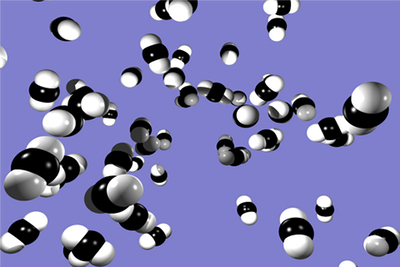### Kinetic Theory of GasesThe kinetic theory of gases attempts to explain certain properties of gases, such as pressure, temperature, or volume, by taking into account their molecular composition and motion. Basically, the theory asserts that pressure takes place not because of static repulsion between molecules, as was Isaac Newton's theory, but because of collisions that take place between molecules that are moving at different speeds.

Quite simply, Avogadro's number refers to the number of molecules in a mole of any given substance. The actual mathematical value of Avogadro's number is approximately 6.0221415 × 1023. Avogadro's number is used to calculate the results of chemical reactions. It allows chemists to determine the exact amounts of substances produced in a given reaction.

Ideal Gas Law

The Ideal gas law is an equation that represents the state of a hypothetical ideal gas. It is a good approximation to the activities of many gases under many conditions, although it does have several limitations. The state of an amount of gas is determined by its pressure, volume, and temperature. The modern form of the equation is: PV = nRT, where p is the absolute pressure of the gas; V is the volume of the gas; n is the amount of substance of the gas, usually measured in moles; R is the gas constant (which is 8.314472 JK-1mol-1 in SI units); and T is the absolute temperature. Since it fails to acknowledge both molecular size and intermolecular attractions, the ideal gas law is most accurate for monoatomic gases at high temperatures and low pressures. The neglect of molecular size becomes less crucial for larger volumes, i.e., for lower pressures.

### Subscribe Now!

Subscribe to our Free Experiment of the Month Club

*
*
*